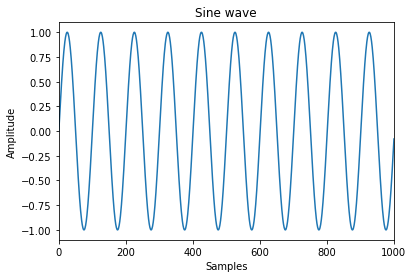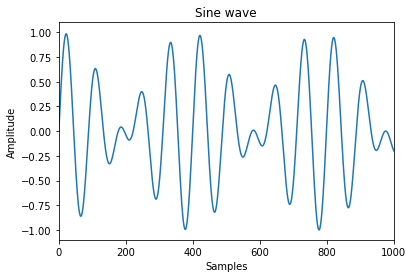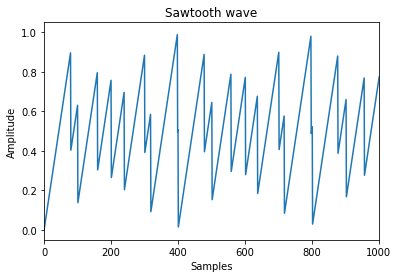## The state of artificial intelligence

A nice summary on the current state of AI and machine learning applications by Professor Andrew Ng, the former chief scientist at Baidu, where he led the company’s Artificial Intelligence Group.

## TensorSynth, how to make music with TensorFlow

As a musician and datascience lover, I was wondering if I could use the Google TensorFlow machine learning framework to actually make music.

Musical tones are actually sine waves, stacked on top of each other. So, if I could produce those waves in Tensorflow, stack them and play them in a musical order, music should come out of Tensorflow!

For this to work, I had to take several steps:

1. Produce a sine wave using Tensorflow and output it as a wav file to listen to
2. Combine multiple sine waves into polyphonical sounds
3. Play music, by letting a midi file define the notes to by played

Now, let’s make some music!

## Step 1: Produce a sine wave using Tensorflow and output it as a wav file

We start by importing the TensorFlow framework as tf and the math library of python (for the math.pi constant).

Our first example is a pattern of notes that we want to play. We define a vecotr having 1.0 when the note should sound and 0.0 when not note should sound. This is the ‘example’ vector.

Then, we define the step_duration which defines for every value in the ‘example’ vector, how long this step takes. In our case, this is 100ms.

For the eventual wav file we’re going to produce, we need a sample frequency. This is the smallest time step for our signal. In this case it’s 44.1 KHz.

Then, we convert the example vector into samples. Essentially we blow up the vector to contain ‘samples’. For this, we calculate the samples_per_step, which contain the number of samples for each value in the ‘example’ vector. Now we end up with a much larger vector called example_samples which contain the actual samples.

Now we apply the sine wave on the values of the example_samples by multiplying the sine wave with the values of the example_samples. Note that the example_samples contain the gain of the sine wave. To be able to show the graph, we also create the x range.

Finally, from the y vector, we let TensorFlow create a wav file.

In :
```import tensorflow as tf
import math as math
import matplotlib.pyplot as plt
from tensorflow.contrib import ffmpeg

example = tf.constant([1.0, 1.0, 1.0, 0.0, 1.0, 0.0, 1.0, 0.0, 1.0, 1.0, 1.0, 1.0, 0.0, 0.0])
step_duration = tf.constant(0.1) # 100ms
sample_freq = tf.constant(44100.0) # 44.1 kHz
samples_per_step = tf.cast(tf.multiply(sample_freq, step_duration), tf.int32) # sample_freq*step_duration

# Now, expand the example vector into example_samples using the TensorFlow 'tile' function
def expand(input, amount):
input_2d = tf.reshape(input, [tf.shape(input), 1])
input_2d = tf.tile(input_2d,[1,amount])
return tf.reshape(input_2d,[tf.size(input_2d)]) # Make 1D again

example_samples = expand(example, samples_per_step)

def sin_wave(x, frequency):
period = tf.divide(frequency,sample_freq)
period = tf.multiply(period,tf.constant(math.pi*2))

x_normalized = tf.multiply(x, period)
# y = sin(x_n)
y=tf.sin(x_normalized)
return y

# Create an x for the timeline, in samples
x = tf.range(0.0, tf.size(example_samples), 1)

# The y is the actual sine wave in this case with a frequency of 440.0 Hz
y = tf.multiply(sin_wave(x, 440.0), example_samples)

# Generate an audio file from the waveform (1d vector)
def generate_wav_file(filename, input_waveform):
audio_input = tf.expand_dims(input_waveform, 1) # expand because multiple channels supported
uncompressed_binary = ffmpeg.encode_audio(
audio_input, file_format='wav', samples_per_second=tf.cast(sample_freq, tf.int32))
return tf.write_file(tf.constant(filename),uncompressed_binary)

with tf.Session() as sess:
x_result, y_result, wav_result = sess.run([x,y, generate_wav_file('output.wav', y)])

# Plot the first 1000 samples
plt.plot(x_result, y_result)
plt.title("Sine wave")
plt.ylabel("Amplitude")
plt.xlabel("Samples")
plt.xlim(0.0,1000.0)
plt.show()

from wavplayer import WavPlayer
WavPlayer('output.wav').show()
```Simple Test

## Combine multiple sine waves into polyphonical sounds

Now that we’re able to create one sine wave, let’s create more, sounding together.

For this, our ‘example’ vector will not be 1D anymore but 2D, because we need more notes. Every row will be a note. Let’s define the vector as having the note frquency as the first value on each row, following with 1.0 and 0.0 to indicate if the note is sounding or not.

In :
```# The example vector will now have 2 notes. The first value of every row is the frequency.
example = tf.constant([
[440.0, 1.0, 1.0, 1.0, 0.0, 1.0, 0.0, 1.0, 0.0, 1.0, 1.0, 1.0, 1.0, 0.0, 0.0],
[553.0, 1.0, 1.0, 1.0, 0.0, 1.0, 1.0, 1.0, 0.0, 0.0, 0.0, 1.0, 0.0, 1.0, 0.0]
])

def create_monophonic_audio(frequency, input):
example_samples = expand(input, samples_per_step)

# Timeline in samples
x = tf.range(0.0, tf.size(example_samples), 1)
y = tf.multiply(sin_wave(x, frequency), example_samples)

return y

def create_polyphonic_audio(input):
signals = tf.map_fn(
# The first element is the frequency, the rest the gains
lambda x: create_monophonic_audio(x, tf.slice(x, , tf.shape(x)-1)),
input)

# Mix the audio signals
sum = tf.reduce_sum(signals, axis=0)
return tf.divide(sum, tf.reduce_max(sum))

# The polyphonic audio
y = create_polyphonic_audio(example)

# Timeline for the graph
x = tf.range(0.0, tf.size(y), 1)

with tf.Session() as sess:
x_result, y_result, wav_result = sess.run([x, y, generate_wav_file('output2.wav', y)])

# Plot the first 1000 samples
plt.plot(x_result, y_result)
plt.title("Sine wave")
plt.ylabel("Amplitude")
plt.xlabel("Samples")
plt.xlim(0.0,1000.0)
plt.show()

from wavplayer import WavPlayer
WavPlayer('output2.wav').show()
```Simple Test

A sin wave sounds a little boring. By introducing a sawtooth wave, our audio starts to sound much better.

In :
```def sawtooth_wave(x, tone_freq):
period = tf.divide(tone_freq,sample_freq)

x_normalized = tf.multiply(x,period)
# y = x*period - floor(x*period)
y = tf.subtract(x_normalized, tf.floor(x_normalized))
return y

def create_monophonic_audio(frequency, input):
example_samples = expand(input, samples_per_step)

# Timeline in samples
x = tf.range(0.0, tf.size(example_samples), 1)
y = tf.multiply(sawtooth_wave(x, frequency), example_samples)

return y

# The polyphonic audio
y = create_polyphonic_audio(example)

# Timeline for the graph
x = tf.range(0.0, tf.size(y), 1)

with tf.Session() as sess:
x_result, y_result, wav_result = sess.run([x, y, generate_wav_file('output3.wav', y)])

# Plot the first 1000 samples
plt.plot(x_result, y_result)
plt.title("Sawtooth wave")
plt.ylabel("Amplitude")
plt.xlabel("Samples")
plt.xlim(0.0,1000.0)
plt.show()

from wavplayer import WavPlayer
WavPlayer('output3.wav').show()
```Simple Test

## Play music, by letting a midi file define the notes to by played

Actually this is not done in TensorFlow. We use the Python midi module by Giles Hall to convert a midi file into the ‘example’ vector as shown above.

A midi file is just a simple file containing events. Every event tells something about an occurence. The occurrences we are interested in are if a note should be played or not (NoteOn and NoteOff events). These represent exactly the 1.0s and the 0.0 in our ‘example’ vector.

A midi file contains multiple tracks having those events, so we should all process them.

Another challenge is to convert a midi note into the right frequency. Midi notes are defined as numbers, ranging from 0 to 127. The 440.0 Hz note (A) is define as note number 69 in midi. So with a simple formula, we can transform a midi note value into a frequency:Just as in audio signals where we’re dealing with a sample frequency, in midi we’re dealing with ‘ticks’. A tick is the smallest note duration. A midi file defines a ‘resolution’ which is in pulses per quarter note (PPQ). From this resolution, and the tempo (which we manually define to be 120BPM here), we can calculate the ‘tick’ duration.

As a first step we create a matrix of 127 rows. Every row represent a note. We put a 1.0 if a note is on and a 0.0 if a note is off. For every tick a value is created. We do this in the get_key_matix() function, for every midi track.

Then, for every row in the matrix, we know the frequency. It is defined by the row index, e.g. row 69 is 440.0 Hz, see the formula above. We insert this frequency as the first value of every row. Now we have exactly the ‘example’ vector of step 2. We do this in get_ts_input_data(). Note that, if a row contains only 0.0s, we can leave out the row because it never plays a note. By removing it, we reduce computation time later in TensorFlow.

It’s also important that every row contains the same number of values. Because the different tracks in the midi file can differ in length, have have to adjust this.

Finally we set the new step_duration and samples_per_step and recalculate our audio using TensorFlow. Enjoy the music!

In :
```import midi

# Read in the midi file, in our case minuet in G by J.S. Bach

# Get the resolution and, based in 120 BPM, calculate the tick_duration is seconds
resolution = pattern.resolution  #ppq (pulse per quarter)
tempo = 500000.0  # In us per quarter note (so 120BM is 500000)
tick_duration = (tempo / resolution) / 1e6  # in seconds

# Define the function to convert a midi note value to a frequency
def get_frequency(midi_note_value):
return 440.0 * (2**((midi_note_value-69)/12.0))

# And create a matrix for a midi track
def get_key_matrix(track):
keys = []
output = []
for key_index in range(0, 127):
keys.append(0.0)
output.append([])

for event in track:
is_end_of_track = event.name == 'End of Track'
if event.tick != 0 or is_end_of_track:
# Now produce output
ticks = event.tick

# Copy the keys current state into the output buffer, ticks times
for _ in range(0, ticks):
key_index = 0
for key_value in keys:
if is_end_of_track:
# Make sure everything is silent in the end
output[key_index].append(0.0)
else:
output[key_index].append(key_value)
key_index = key_index+1
pass

if event.name == 'Note On':
midi_note = event.data
velocity = event.data

if velocity == 0:
keys[midi_note] = 0.0 # A Note On with velocity 0.0 should be treated as a Note Off
else:
keys[midi_note] = 1.0

if event.name == 'Note Off':
midi_note = event.data

keys[midi_note] = 0.0

return output

# Get the TensorFlow input data. Put the frequency as the first value of every row and leave out
# rows that do not produce sounds
def get_ts_input_data(track):
matrix = get_key_matrix(track)

# Insert the frequency as the first item in each row
row_index = 0
for row in matrix:
midi_note = row_index
frequency = get_frequency(midi_note)
row.insert(0, frequency)
row_index = row_index + 1

# Get all distinct notes
applicable_notes = set([event.data for event in track if event.name == 'Note On'])

# Remove all rows that have no note in the midi track
matrix_with_applicable_notes = []
row_index = 0
for row in matrix:
if row_index in applicable_notes:
matrix_with_applicable_notes.append(row)
row_index = row_index+1

return matrix_with_applicable_notes

# For all tracks in the midi file, get the input data and create one big matrix
# which is the input for our TensorFlow stuff from the earlier steps
ts_input1 = get_ts_input_data(pattern)
ts_input2 = get_ts_input_data(pattern)

# Make sure all inputs have the same length
inputs = [ts_input1, ts_input2]
max_length = max([len(inp) for inp in inputs])
for input in inputs:
for row in input:
for _ in range(0, max_length-len(row)):
row.append(0.0)

ts_input = []
ts_input.extend(ts_input1)
ts_input.extend(ts_input2)

example = tf.constant(ts_input)
filename = 'minuet in g.wav'

# The step_duration of our input is now defined by the tick_duration of the midi file
step_duration = tick_duration
samples_per_step = tf.cast(tf.multiply(sample_freq, step_duration), tf.int32) # sample_freq*step_duration

with tf.Session() as sess:
wav_result = sess.run(generate_wav_file(filename, create_polyphonic_audio(example)))

from wavplayer import WavPlayer
WavPlayer(filename).show()
```

Simple Test